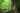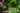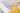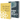# const vs let, and var in JavaScript

by Robin WieruchThere are three different ways to declare a variable in JavaScript: const, let and var. Historically, var has been the only way of :

`var name = 'Robin Wieruch';`

An addition to JavaScript -- to be specific: JavaScript ES6 in 2015 -- has made `const` and `let` available to the language:

`let firstName = 'Robin'; const lastName = 'Wieruch';`

Obviously more options on how to declare and define a variable in JavaScript doesn't make it easier for developers being new to the language. But we can quickly make things easier for beginners: One can say that const and let took over and var isn't really used anymore in modern JavaScript. It's mainly because of two reasons:

• const and let are more specific about their usage and intention
• var comes with its quirks which have been addressed with const and let

## let vs var

There are two reasons I can think of why let (and const) is(/are) superior to var: hoisting and scoping. Let's take the following example code for summing up the age of an array of persons with var as the variable declaration of choice:

`var personOne = {  name: 'Robin Wieruch',  age: 30,}; var personTwo = {  name: 'Liesa Huppertz',  age: 29,}; var family = [personOne, personTwo]; function sumAge(persons) {  var sum = 0;   for (var i = 0; i < persons.length; i++) {    sum = sum + persons[i].age;  }   return sum;} var result = sumAge(family); console.log(result);// 59`

First, hoisting has been an issue with var, because every variable declared with var is initialized with undefined by default even though it hasn't been declared/defined yet in the actual code:

`function sumAge(persons) {  console.log(sum);  // undefined   var sum = 0;   for (var i = 0; i < persons.length; i++) {    sum = sum + persons[i].age;  }   return sum;}`

If our variable wouldn't be declared in the code eventually, we would get a "ReferenceError: sum is not defined" once our JavaScript code executes, which may already be the desired behavior anyway. However, since sum is declared/defined somewhere below in the code, JavaScript preventively initializes it as undefined.

This doesn't seem to be correct when reading the code though, because one would assume that the variable is declared or defined once it has been actually declared or defined. By using let instead of var, we avoid this behavior and get the desired "ReferenceError" anyway:

`function sumAge(persons) {  console.log(sum);  // ReferenceError: sum is not defined   let sum = 0;   for (var i = 0; i < persons.length; i++) {    sum = sum + persons[i].age;  }   return sum;}`

Second, scoping has been another issue when declaring JavaScript variables with var. Let's take the previous example again, but output another var defined variable:

`function sumAge(persons) {  let sum = 0;   for (var i = 0; i < persons.length; i++) {    sum = sum + persons[i].age;  }   console.log(i);  // 2   return sum;}`

Since var is function-scoped in JavaScript, the iterator of our for-loop is accessible outside of the for-loop (block). In contrast to var, let is block-scoped which means it is only defined in a block such as the for-loop:

`function sumAge(persons) {  let sum = 0;   for (let i = 0; i < persons.length; i++) {    sum = sum + persons[i].age;  }   console.log(i);  // ReferenceError: i is not defined   return sum;}`

Again, this is the more desired behavior established by let (and const) in comparison to var. Having let (and const) being block-scoped, helps us the same way as the hoisting behavior from before, to reason about our code. Without using var, we are doing fine as JS developers just using const and let.

## const vs let

So if only const and let are primarily used in JS, because of the hoisting and scoping issues of variables declared with var, what's the major difference between const and let then? Since both are block-scoped and not hoisted, the only differences are that

• a variable declared with const cannot be re-assigned
• a variable declared with let can be re-assigned
`let firstName = 'Robin';firstName = 'Dennis';// does work const lastName = 'Wieruch';lastName = 'Huppertz';// doesn't work`

Some people may get to the immediate conclusion that a JavaScript variable declared with const must be (unchangeable) then, because it cannot be mutated (changed). However, it's important to know that if const is used for a JavaScript object or array, its internal properties can still be re-assigned:

`const person = {  firstName: 'Robin',  lastName: 'Wieruch',}; person = {  firstName: 'Thomas',  lastName: 'Wieruch',};// doesn't work person.firstName = 'Dennis';// does work console.log(person.firstName);// "Dennis"`

That's why it's not a good idea to mistake JavaScript's const variable declaration with immutable data structures.

However, using const and let gives us stronger signals about the usage of the variable in contrast to var. For instance, const gives us a strong signal -- but just a signal, because it's not enforced for JavaScript for all JavaScript data types as we have seen in the previous example -- that the variable is intended (!) to be immutable. If someone would want to signal the intention of a JavaScript being mutable, then one would use let instead of const for the variable declaration:

`let personOne = {  firstName: 'Robin',  lastName: 'Wieruch',};// Saying: "Hey, it's okay to change this variable eventually." let personTwo = {  firstName: 'Liesa',  lastName: 'Huppertz',};// Saying: "Hey, it's okay to change this variable eventually." function marryHerToHim(her, him) {  her.lastName = him.lastName;} marryHerToHim(personTwo, personOne); console.log(personTwo);// { firstName: 'Liesa', lastName: 'Wieruch' }`

However, since it's a common practice to keep data structures immutable in JavaScript, the previous example would be better expressed without changing one of the objects and instead returning a new object for the desired change. Hence, using const instead of let would signal this intention, but as mentioned before, not enforce it:

`const personOne = {  firstName: 'Robin',  lastName: 'Wieruch',};// Saying: "Hey, don't change this variable over its lifetime." const personTwo = {  firstName: 'Liesa',  lastName: 'Huppertz',};// Saying: "Hey, don't change this variable over its lifetime." function marryHerToHim(her, him) {  return {    firstName: her.firstName,    lastName: him.lastName,  };}// Saying: "Instead return a new variable if the variable has to change." const marriedPersonTwo = marryHerToHim(personTwo, personOne); console.log(marriedPersonTwo);// {//   firstName: "Liesa",//   lastName: "Wieruch",// }`

So if const is used most often, because it doesn't give you the ability to re-assign variables, when should one use let instead of const? Commonly let is used in operations where variables have to change by necessity:

`const personOne = {  name: 'Robin Wieruch',  age: 30,}; const personTwo = {  name: 'Liesa Huppertz',  age: 29,}; const family = [personOne, personTwo]; function sumAge(persons) {  let sum = 0;   for (let i = 0; i < persons.length; i++) {    sum = sum + persons[i].age;  }   return sum;} const result = sumAge(family); console.log(result);// 59`

In for-loops it's common to see let for the iterating variable. Also if a variable goes through transformations over time, which is the case for the sum of the age here, it has to be defined as let, because otherwise we would run into a Uncaught TypeError: Assignment to constant variable.-exception:

`function sumAge(persons) {  const sum = 0;   for (let i = 0; i < persons.length; i++) {    sum = sum + persons[i].age; // doesn't work  }   return sum;} const result = sumAge(family); // Uncaught TypeError: Assignment to constant variable.`

So we can see how there are clear lines between using const vs let in JavaScript. If we want to embrace the intention of keeping immutable data structures by not re-assigning them, and by avoiding to re-assign their internal properties, const gives us and others in a code base a pretty strong signal to not change the variable. Instead, if we want to change a variable, in fluctuant operations like for-loops, we can use let instead of const.

### Exercises:

As a rule of thumb, my recommendation would be:

• avoid var, because of its weird issues with scoping/hoisting
• use const as a default (signals variable shouldn't change)
• use let when variable should be re-assigned## JavaScript Variable Tutorial for Beginners

In every programming language, you will find variables. This also holds true for JavaScript. Essentially variables are used to carry information. Without them, it wouldn't be really possible to code…## How to Webpack 5 with Babel - Setup Tutorial

Babel enables one writing code with JavaScript features that aren't supported by most browser yet. Perhaps you have heard about JavaScript ES6 (ES2015) , ES7, and other versions of ECMAScript…